Let's review...

How many ways can you arrange the letters in this word?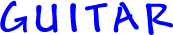It's just like books -- 6 letters...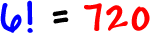But, what if some letters are repeated?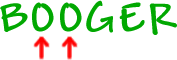The problem is that these letters are the same.  So, we can't tell the difference between these two arrangements:and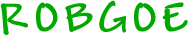which O is which O?

The answer is that it doesn't matter!

So, when we figure this guy out, we have to divide out that double "O" problem.

Check it out:

To arrange 6 letters is 6!

To arrange the two O's would be 2!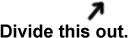So, the number of ways to arrange the letters in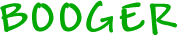is